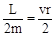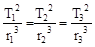# Gravitation

#### Kepler Laws

Kepler found important regularities in the motion of the planets. These regularities are known as Kepler's three laws of planetary motion.
First Law :
Every planet move around the sun in an elliptical orbit with sun at one of the focus. This is the law of orbits.
Second Law :
The line joining the sun to the planet sweeps out equal area in equal interval of time i,e. the area velocity is constant. This is the law of area. The second law tells us that the planet will move most slowly when it is farthest from sun and most rapidly when it is nearest to sun. So we can say that this law is similar to law of conservation of angular momentum. Where
L = angular momentum
m = mass of planet
v = Linear velocity component ⊥ to r
r = Distance of sun & planet.Areal velocity =Conclusions from the figure :
If a planet moves from A to B in time t1, and from C to D in time t2, then if
t1 = t2, Area of SBA = Area of SCD.
t1 > t2, Area of SBA > Area of SCD.
t1 < t2, Area of SBA < Area of SCD.
NOTE : When the planet is nearest to the sun, its speed is maximum and when it is farthest form the sun, then its speed is minimum.Third Law : The square of the period of revolution (T) of any planet around the sun is directly proportional to the cube of its average distance (r) from the sun. This is the law of periods.
T2 ∝ r3 or T2 = kr3, where k = constant. Thus, it is clear from the law that larger the distance of a planet from the sun, larger will be its period of revolution around the sun.
NOTE :
(a) Mercury is the nearest planet to the sun and its time period is 88 days while Pluto is the farthest planet and its time period is 248 years.
(b) Kepler's laws are valid for motion of satellites.
(c) For all the planets of the sun.= ... = constant
(d) When a planet comes close to the sun its linear velocity increases.

If you want to give information about online courses to other students, then share it with more and more on Facebook, Twitter, Google Plus. The more the shares will be, the more students will benefit. The share buttons are given below for your convenience.

#### IIT (Class X)

• Unit, Dimension & Error
• Vectors
• Motion in One Dimension
• PROJECTILE MOTION
• NEWTON'S LAWS OF MOTION & FRICTION
• WORK, POWER, ENERGY & CONSERVATION LAWS
• CIRCULAR MOTION & ROTATIONAL DYNAMICS
• GRAVITATION